# For a system, H2(g) + I2(g) ⇌ 2 HI(g), Kc = 62.9 at 750 K. 2.80 moles of HI were placed in a 10.0-liter container, brought up to 750 K, and allowed to come to equilibrium. Which situation described below is true, at equilibrium?a. [HI] = 2 × [H2] b. [HI] = [H2] c. [HI] < [H2] d. [HI] > [H2] e. [H2] > [I2]

Question
12 views

For a system, H2(g) + I2(g) ⇌ 2 HI(g), Kc = 62.9 at 750 K. 2.80 moles of HI were placed in a 10.0-liter container, brought up to 750 K, and allowed to come to equilibrium. Which situation described below is true, at equilibrium?

a. [HI] = 2 × [H2] b. [HI] = [H2] c. [HI] < [H2] d. [HI] > [H2] e. [H2] > [I2]

check_circle

Step 1

The reverse reaction can be written as shown below.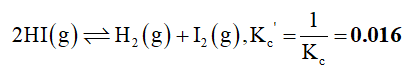The expression for equilibrium constant for the above reaction can be written as shown below,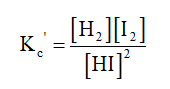Step 2

Given that, the initial number of moles of HI is 2.80 mol. The volume of the container is 10.0 L. The initial concentration of HI can be calculated as given below.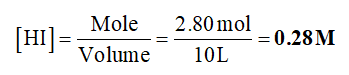An equilibrium table can be set up as given below.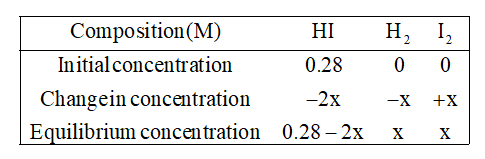Now, these values in the fourth row can be inserted in the equilibrium constant expression as shown below.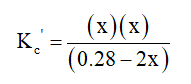Now, the above equilibrium expression can be solved for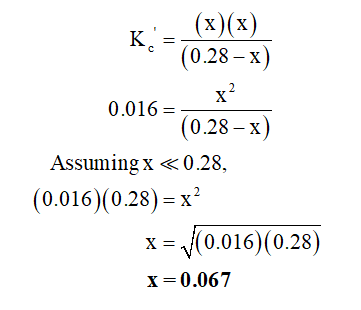...

### Want to see the full answer?

See Solution

#### Want to see this answer and more?

Solutions are written by subject experts who are available 24/7. Questions are typically answered within 1 hour.*

See Solution
*Response times may vary by subject and question.
Tagged in